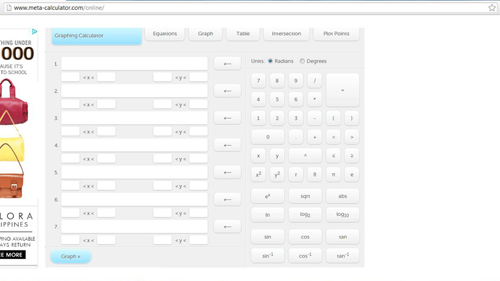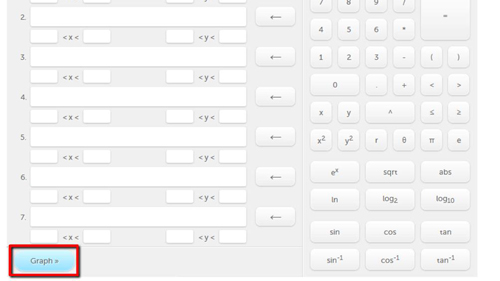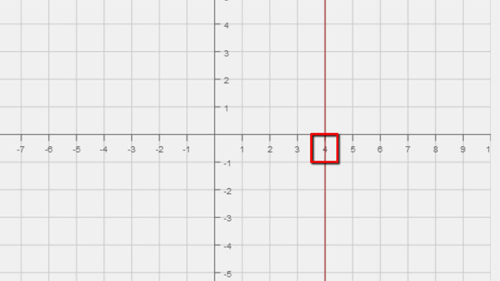## How to Use Graphing Calculator

In this tutorial you are going to learn how to use a graphing calculator online which can be a great alternative to using one of the expensive physical models.

Step # 1 – Using the Graphic Calculator

First you are going to need to visit the site that has an online graphic calculator. Navigate to “http://www.meta-calculator.com/online” and you will see a large calculator interface which will be familiar to anyone who has had to use one of these calculators before.Step # 2 – Entering a Simple Equation

Of course, this type of calculator is capable of some very advanced mathematics but for this example we are just going to plot a very simply equation. Into the first box on the left we are going to enter the equation “1+x=5” and then click “Graph” at the bottom of the screen.Step # 3 – Viewing the Graphical Results

A new screen will load and you will see that the equation has been plotted on the graph – as you can see it has correctly revealed the answer as “4”. If you want to go back and solve another equation simple click the “Go back” button and you will see the previous screen. That is essentially how to use a graphing calculator and as you can see it is a great alternative to having a physical device.